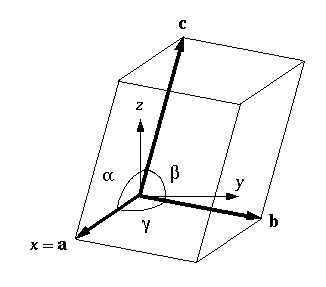# Crystallography

## 1. Crystal structure and periodicity:

A crystal structure is defined as a regular arrangement of atoms decorating a periodic, three dimensional lattice. the lattice is defined as the set of points which is created by all integer linear combinations of three basis vectors a, b and c. In other words, the lattice T is the set of all vectors t of the form:

t = ua + vb + wc               ( where u,v,w are arbitrary integers)

Such a lattice of building blocks is called a Bravais lattice. Auguste Bravais was the first person who listed the 14 space filling lattices and 5 plane filling lattices in 1850. So they called named after him.

The 14 space filling three-dimensional lattices are divided into 7 crystal systems. Namely: (1) cubic, (2) tetragonal, (3) orthorhombic, (4) rhombohedral, (5) hexagonal, (6) monoclinic, and (7) triclinic.Fig 1.1 A diagram of the 14 Bravais lattices in 3D

The angles between the basis vectors are represented by the Greek letters α, β and γ ; the six numbers {a,b,c,α,β,γ} are known as the lattice parameters of the unit cell. The lattice parameters can be used to distinguish between the seven crystal systems. It is a basic property of a lattice tha all lattice sites are equivalent. In other words, any site can be selected as the origin.Fig 1.2 Schematic representation of a general unit cell

## 2. The reciprocal lattice:

Miller indices are used to label crystal planes with the familiar (hkl) notation based on their intercepts with the crystallographic reference axes. As for directions, negative indices are often indicated by a bar or minus sign written above the corresponding index, eg. (00).  Every crystal has two lattices associated with it, a crystal lattice and a reciprocal lattice. A diffraction pattern of a crystal is a map of the reciprocal lattice of the crystal.

The reciprocal basis vectors can be derived from the following definition:

ai . aj* ≡ δij         ( where δij is the Kronecker delta)

This definition then leads to expressions for the reciprocal basis vectors:

a* = (b x c) /a . (b x c)

b* = (c x a ) / a . (b x c)

c* = (a x b) / a . (b x c)

The reciprocal lattice vector g , with components (h,k,l) is perpendicular to the plane with Miller indices (hkl), so often denoted as ghkl:

ghkl = ha* + kb* + lc*     and    |ghkl | = 1/dhkl        ( where dhkl  is the spacing between lattice planes)

3.Crystal diffraction conditions: